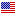1st Edition

# Optimal Control Weakly Coupled Systems and Applications

ISBN 9780849374296
320 Pages 19 B/W Illustrations
Published December 10, 2008 by CRC PressFree Shipping (6-12 Business Days)
shipping options

USD \$210.00
ISBN 9781315221786
320 Pages 19 B/W Illustrations
Published October 3, 2018 by CRC Press

Also available as eBook on:

Unique in scope, Optimal Control: Weakly Coupled Systems and Applications provides complete coverage of modern linear, bilinear, and nonlinear optimal control algorithms for both continuous-time and discrete-time weakly coupled systems, using deterministic as well as stochastic formulations. This book presents numerous applications to real world systems from various industries, including aerospace, and discusses the design of subsystem-level optimal filters. Organized into independent chapters for easy access to the material, this text also contains several case studies, examples, exercises, computer assignments, and formulations of research problems to help instructors and students.

Preface
Introduction
References

Recursive Approach for Linear Weakly Coupled Control Systems
Linear Weakly Coupled Control Systems.
Introduction
Weakly Coupled Linear Continuous Systems
Approximate Linear Regulator for Continuous Systems
Weakly Coupled Linear Discrete Systems
Approximate Linear Regulator for Discrete Systems
Output Feedback Control for Linear Weakly Coupled Systems
References
Quasi-Weakly Coupled Linear Control Systems
Optimal Controller for Quasi-Weakly Coupled Linear Systems
Reduced-Order Controller for a Class of Weakly Coupled Systems
Notes
Appendix 3.1
References
Weakly Coupled Singularly Perturbed Systems
Introduction
Weakly Coupled Singularly Perturbed Linear Control Systems
Quasi-Weakly Coupled Singularly Perturbed Control Systems
Conclusion
References
Decoupling Transformation, Lyapunov Equation, and Boundary Value Problem
Decoupling Transformation of Gaji_c and Shen
Decoupling Transformation for N Weakly Coupled Subsystems
Decompositions of the Differential Lyapunov Equation
Boundary Value Problem of Linear Continuous Systems
Boundary Value Problem of Linear Discrete Systems
References
Stochastic Linear Weakly Coupled Systems
Continuous Weakly Coupled Stochastic Linear Control Systems
Discrete Weakly Coupled Stochastic Linear Control Systems
Stochastic Output Feedback of Discrete Systems
Optimal Control of Stochastic Jump Parameter Linear Systems
References
Nash Differential Games
Solution of Coupled Algebraic Riccati Equations
Numerical Example
Appendix 7.1
Appendix 7.2: Algorithm for Solving Coupled Algebraic Riccati Equations of Nash Differential Games
References

Hamiltonian Approach for Linear Weakly Coupled Control Systems
Finite Time Optimal Control via Hamiltonian Method
Open-Loop Optimal Control in Continuous-Time
Open-Loop Optimal Control in Discrete-Time
Differential Riccati Equation
Difference Riccati Equation
Concluding Remarks
Appendix 8.1
Appendix 8.2
References
Hamiltonian Method for Steady State Optimal Control and Filtering
Exact Decomposition of the Weakly Coupled Continuous-Time Algebraic Riccati Equation
Optimal Filtering in Continuous-Time
Optimal Control and Filtering in Discrete-Time
Optimal Control of Weakly Coupled Systems with N Subsystems
Conclusion
Appendix 9.1
References
Eigenvector Method for the Hamiltonian Approach
Introduction
Decomposition of Weakly Coupled Algebraic Riccati Equation
Eigenvector Method for Nonsymmetric (Nonsquare) Algebraic Riccati Equation
Exact Decomposition Algorithm for Weakly Coupled Systems
Examples
Conclusion
Appendix 10.1 Justification of Step 3 of Algorithm 10.2
Appendix 10.2 On the Number of Solutions to Nonsymmetric ARE
References

Bilinear Weakly Coupled Control Systems
Optimal Control of Bilinear Weakly Coupled Systems
Introduction
Optimal Control for Weakly Coupled Bilinear Systems Using SGA
Robust H1 Control for Weakly Coupled Bilinear Systems with Parameter Uncertainties Using SGA
Conclusion
References
Index

We offer free standard shipping on every order across the globe.